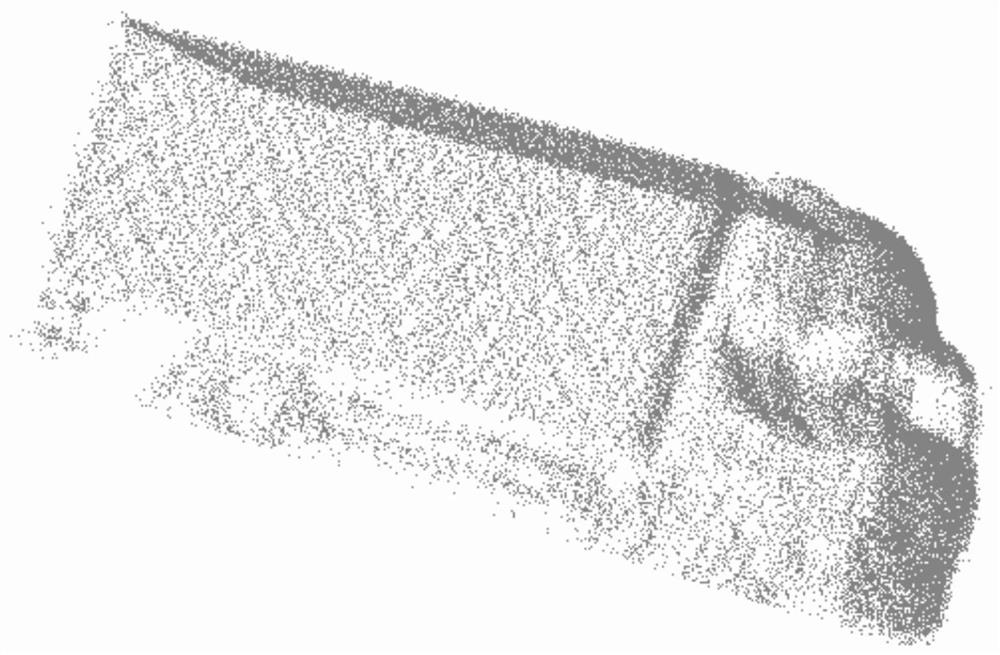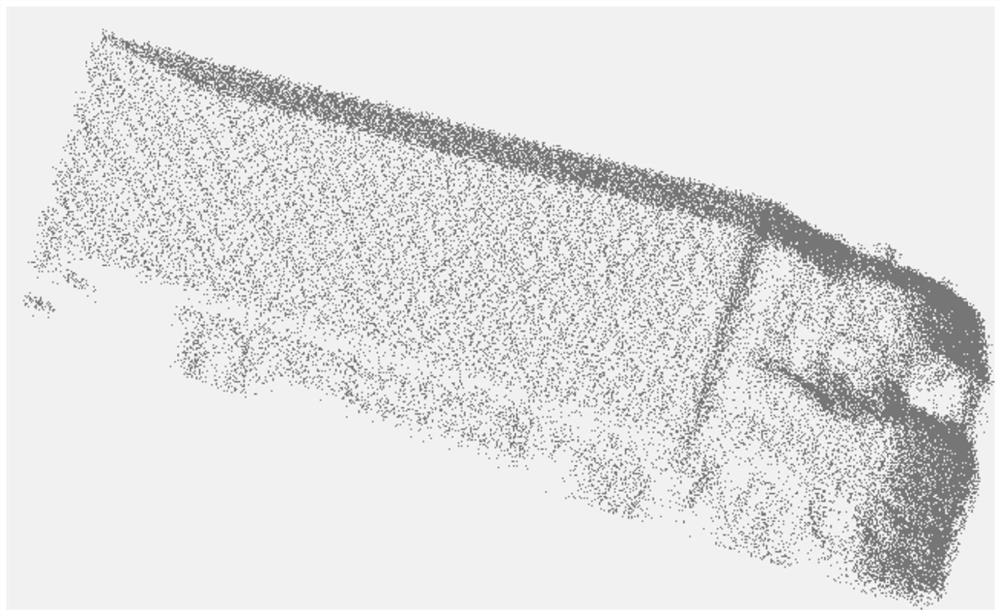# Automobile radar point cloud data processing method

## A technology for point cloud data and automotive radar, which is applied in the processing field of automotive radar point cloud data and can solve problems such as complex computing

Pending Publication Date: 2022-03-01

5 Cites 0 Cited by

## AI-Extracted Technical Summary

### Problems solved by technology

 In order to maintain the invariance of different arrangements of data, the methods currently used in the literature include: (1) reordering the unordered data, this method does not directly process the three-dimensional point cloud data, but through projection and other ...
View more

### Method used

The alignment of point cloud data is to obtain transformation matrix by transformation matrix T (T-Net structure), and this transformation matrix and input point cloud data are multiplied and realized, to guarantee the invariance of model to specific space transformation. Among them, the initial value of the transformation matrix T is a random value, but during the iterative training process of the neural network, due to the constraints of ...
View more

## Abstract

The invention provides an automobile radar point cloud data processing method, which comprises the following steps: S1, marking point cloud original data, selecting a corresponding axle number according to different automobile types, taking one part of the marked point cloud data as training data, and taking the other part of the marked point cloud data as test data; s2, performing model training by using the training data in the S1, firstly aligning the point cloud data and performing deep learning iteration training, then aggregating the extracted feature information, and simultaneously performing deep learning iteration training to obtain a trained model; s3, performing model reasoning by using the test data in the S1 and adopting the model trained in the S2, and comparing the predicted axle type output by the model with the marked truth value to obtain a correct rate; and S4, if the accuracy is greater than the set target accuracy, passing, and if the accuracy is less than or equal to the set target accuracy, increasing the number of the marked point cloud data, and circulating the steps S1-S4 until the accuracy is greater than the set target accuracy.

Application Domain

Character and pattern recognitionNeural architectures +2

Technology Topic

EngineeringData processing +6

## Image

•••## Examples

• Experimental program(1)

### Example Embodiment

 Example 1:
 Treatment method for automobile radar point cloud data, such as Figure 1 - Figure 6 As shown, the step of: S1, the point cloud raw data is labeled, and according to the different models, the number of points after the label is selected as the training data, and the other is used as the test data; S2, the training data of S1 Making model training, first alignment of point cloud data and depth learning iterative training, then polymerizing the extracted feature information, and performing deep learning iterative training to obtain a well-trained model; S3, using S1 test data adopts S2 training Model is performed, compares the predicted axle type of the model output to the true value of the label; S4, the correct rate is greater than the set target accuracy, the correct rate is less than or equal to the set target accuracy Increase the number of points cloud data, loop step S1-S4 until the correct rate is greater than the set target accuracy.
 Putting different models in accordance with their own models, the 4/5 data is divided into training data, 4/5 data divided into training data, and the remaining 1 The data of / 5 is divided into test data. After the detection tracking and cluster of radar point cloud data, get our input: one frame or multi-frame, one car of a car, then passed through a random downsample, the number of points clouds in different objects is random Take 2500 points, get 2500 * 3 Tensor, where 2500 represents the number of points clouds, 3 corresponds to the XYZ coordinates, and the point cloud data after the descending sample is input as the model input data.
 The alignment of point cloud data is to obtain a conversion matrix through the transformation matrix T (T-NET structure), and multiplied the conversion matrix and the input point cloud data to ensure the invariance of the model to a particular spatial conversion. Wherein, the initial value of the transform matrix T is a random value, but during the negative network iterative training, the T matrix training will learn the most conducive to the final classification of transformation due to the constraint of the neural network, such as rotating the point cloud to the front. The T matrix is ​​used in the input data and the first layer feature, and the size is 3x3 and 64x64. The second T matrix considers the addition of the regular item due to too many parameters, so that it is close to the orthogonal matrix, and the information loss of the point cloud is reduced.
 The partial features and global features are connected in series to aggregate information. After the characteristics of each point cloud data is performed by multiple activation (MLP), the features are aligned with one transformation matrix T. Specifically, characteristic is performed by three convolution + batches + activation (MPL), and then the activated Tensor multiplies the characteristic alignment by the matrix obtained by T-Net. Using the MaxPooling this symmetric function algorithm to extract the characteristics of the point cloud data, the maximum pillization operation is performed on the respective dimensions of the feature, and the maxpooling here is the second dimension of the 500 * 1022 Tensor. Take the largest value to get 2500 * 1 Tensor. In the end, by using the global feature of activation (MLP) output 1 * 5 Tensor, 1 * 1024 Tensor passes three convolution + batch returns + activation (MPL), resulting in 1 * 5 matrix, resulting in the final classification score, The maximum axis category of the score is the number of axes of the predicted axle. Neural network iterative training has been taken in 1000 wheels with a model weight file.
In the model of the obtained weight file, enter the test data for S1 in the S1, which output is the type of axle type, which is compared with the true value of the label, and performs the correct percentage statistics, prediction and true value results. Remember 1, otherwise 0, the score of each data is removed by the total number of tests to get the correct rate. Determine whether the correct rate is greater than the target accuracy of our set, the correct rate is greater than or equal to the set target accuracy, the project is over, for example, the correct rate is 99%, then the correct rate> 99% passed, otherwise it will not pass The correct rate is less than or equal to the set target accuracy, increase the number of points cloud data of the label, and the loop step S1-S4 until the correct rate is greater than or equal to the set target accuracy.

## PUM## Description & Claims & Application Information

We can also present the details of the Description, Claims and Application information to help users get a comprehensive understanding of the technical details of the patent, such as background art, summary of invention, brief description of drawings, description of embodiments, and other original content. On the other hand, users can also determine the specific scope of protection of the technology through the list of claims; as well as understand the changes in the life cycle of the technology with the presentation of the patent timeline. Login to view more.
Who we serve
• R&D Engineer
• R&D Manager
• IP Professional
Why Eureka